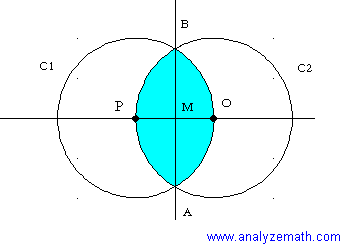# Overlapping Circles Problem

Overlapping circles area problems with detailed solutions.

## Problem

The distance between the centers of two circles C1 and C2 is equal to 10 cm. The circles have equal radii of 10 cm. O is the center of C2 and P is the center of C1. Find the overlapping area of the two circles. Approximate your answer to one decimal place.Solution to Problem

The overlapping area is made up of two equal parts. The idea is to find one part (shown in figure below in blue) then multiply it by 2. Since the two circles have equal radii, M is the midpoint of segment OP. Distance OP = 10 cm so thatd(O,M) = 5 cm
We now use the cosine to find angle BOM.
cos(angle BOM) = d(O,M) / OB = 5 / 10 = 1 / 2
Using arccos function, we obtain.
angle BOM = 60 degrees.
Angle AOB is twice angle BOM, hence.
angle AOB = 120 degrees.
The area in "blue" may be expressed as the difference between area of sector OBPA and area of triangle AOB.
The area of sector OBPA is given by.
sector area = (1 / 2) * r2 * angle BOA
= (1/2) 10 2 (120 * pi / 180)
= 100 pi / 3
The area of triangle OBA is given by.
area of triangle = (1/2) r * r * sin(120 degrees) = 50 sqrt(3) / 2
The area of the "blue" region (Area 1) is given by the difference.
Area 1 = 100 pi / 3 - 50 sqrt(3) / 2
The total overlapping area (Area 2) is twice area 1.
Area 2 = 2(100 pi / 3 - 50 sqrt(3) / 2)
= 122.8 cm 2 (approximated to one decimal place).

## More References and Links to Geometry

Geometry Tutorials, Problems and Interactive Applets.